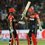# Not true!

Let $a,b,c$ be real numbers such that $a+b+c=0$ and $a^2+b^2+c^2=1.$Prove that $a^2b^2c^2\leq \frac{1}{54}.$When does this equality hold true?Note by Ayush G Rai
5 years ago

This discussion board is a place to discuss our Daily Challenges and the math and science related to those challenges. Explanations are more than just a solution — they should explain the steps and thinking strategies that you used to obtain the solution. Comments should further the discussion of math and science.

When posting on Brilliant:

• Use the emojis to react to an explanation, whether you're congratulating a job well done , or just really confused .
• Ask specific questions about the challenge or the steps in somebody's explanation. Well-posed questions can add a lot to the discussion, but posting "I don't understand!" doesn't help anyone.
• Try to contribute something new to the discussion, whether it is an extension, generalization or other idea related to the challenge.
• Stay on topic — we're all here to learn more about math and science, not to hear about your favorite get-rich-quick scheme or current world events.

MarkdownAppears as
*italics* or _italics_ italics
**bold** or __bold__ bold
- bulleted- list
• bulleted
• list
1. numbered2. list
1. numbered
2. list
Note: you must add a full line of space before and after lists for them to show up correctly
paragraph 1paragraph 2

paragraph 1

paragraph 2

[example link](https://brilliant.org)example link
> This is a quote
This is a quote
    # I indented these lines
# 4 spaces, and now they show
# up as a code block.

print "hello world"
# I indented these lines
# 4 spaces, and now they show
# up as a code block.

print "hello world"
MathAppears as
Remember to wrap math in $$ ... $$ or $ ... $ to ensure proper formatting.
2 \times 3 $2 \times 3$
2^{34} $2^{34}$
a_{i-1} $a_{i-1}$
\frac{2}{3} $\frac{2}{3}$
\sqrt{2} $\sqrt{2}$
\sum_{i=1}^3 $\sum_{i=1}^3$
\sin \theta $\sin \theta$
\boxed{123} $\boxed{123}$

## Comments

Sort by:

Top Newest

Without losing generality we assume that $ab\geq 0$. From the 1st condition we have $a+b=-c$ replace this into the 2nd condition $a^2+b^2+(a+b)^2=1$ By C-S $a^2+b^2\geq\frac{(a+b)^2}{2}$, so $1\geq\frac{3}{2}(a+b)^2 \Rightarrow c^2\leq\frac{2}{3}$. Now we rewrite the 2nd condition $2(a^2+b^2)+2ab=1$ We have this inequality for all reals $a^2+b^2\geq 2ab$, therefore $6ab\leq 1\Rightarrow a^2b^2\leq\frac{1}{36}$ $\therefore a^2b^2c^2\leq\frac{1}{54}$ The equality holds when $(a,b,c)=\bigg(\frac{1}{\sqrt{6}},\frac{1}{\sqrt{6}},\frac{-2}{\sqrt{6}}\bigg);\bigg(\frac{-1}{\sqrt{6}},\frac{-1}{\sqrt{6}},\frac{2}{\sqrt{6}}\bigg)$

- 5 years ago

Log in to reply

Let $\alpha=abc$.

Now, as $0=(a+b+c)^2=a^2+b^2+c^2+2(ab+bc+ca)\\\implies 0=1+2(ab+bc+ca)\\\implies ab+bc+ca=-\frac{1}{2}$

Therefore, $a, b, c$ are the (real) roots of the following function:

$f(x)=x^3-\frac{1}{2}x-\alpha$

For the existence of three real roots, we must have: $f(x_1)f(x_2) \leq 0$ where $x_1$ and $x_2$ are the points of extrema of the function $f(x)$.

Note that $f'(x_1)=f'(x_2)=0$.

Since $f'(x)$ is a quadratic polynamial, it can be solved.

Solving, plugging it into the above inequality, we get: $|\alpha| \leq \frac{1}{3\sqrt6} \\\implies \alpha^2 \leq \frac{1}{54}$

With equality holding if and only if two of the real numbers $a, b, c$ are equal.

Log in to reply

Note: It's slightly more straightforward to just take the cubic discriminant condition for 3 real roots.

Staff - 5 years ago

Log in to reply

The problem was I didn't remeber the cubic discriminant formula then. So, I used calculus in the solution.

Log in to reply

×

Problem Loading...

Note Loading...

Set Loading...